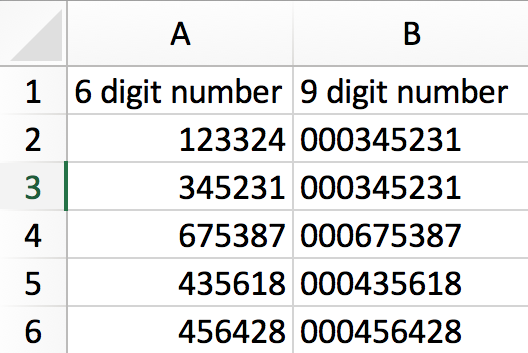# How To Add Zeros To The Beginning Of A Number In Microsoft Excel or Google Sheets

In Google Spreadsheets or Microsoft Excel, you can add zeros to the beginning of a number by using a function.

## Steps

See the example below: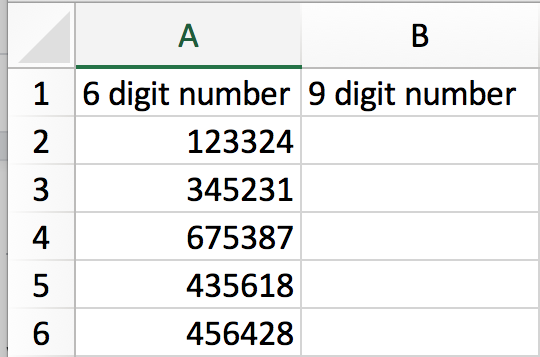All you would need to do is add in a function, “TEXT”, in a new cell.

The function will look like this:

=TEXT(A2,"000000000")

This will make it as many characters long. In this example we wanted the number in “ID” to have one extra number, or change it from four characters to five. In “TEXT” we added five zeros because we wanted one extra zero in the front.

Following the example above:

Step 1.

Select the cell B2 and write the formula: =TEXT(A2,"000000000"). Then press Enter on your keyboard.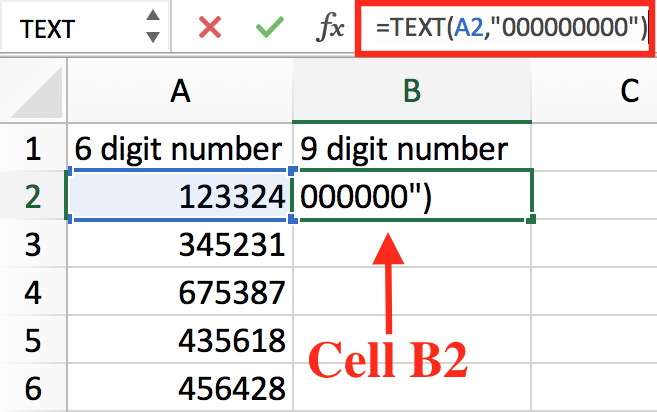Step 2.

The function will return the four digit number with the added zero in front of it.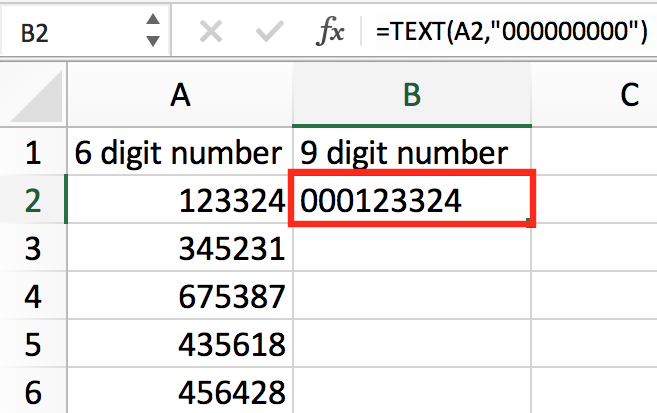Step 3.

Complete Step 1 for the remaining cells (B3 through B6). Changing A2 in the formula to the corresponding row number.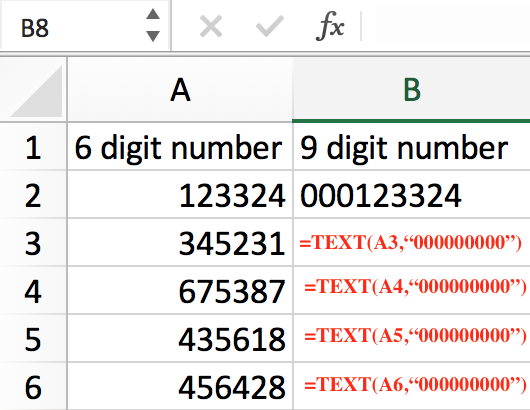Step 4.

The end result should look like the example below.